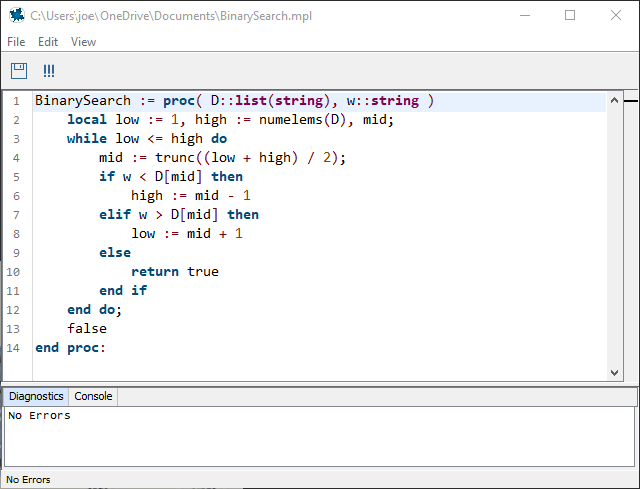Correlation Coefficient - Maple Help

Correlation Coefficient

Main ConceptPearson Product-Moment Correlation Coefficient The Pearson Product-Moment Correlation Coefficient (also known as r) is a value that measures the correlation between two variables $\mathbit{X}$ and $\mathbit{Y}$. The coefficient value ranges from to $1$, where a positive value represents a positive correlation, a value of $0$ represents no correlation and a negative value represents a negative correlation. Values of ±$1$ are considered total correlations and only occur when all of the data points lie on a straight line. Data sets with values of r near 0 are considered to have weak correlations, while data sets with values of r near $+1$ or $-1$ are considered to have strong correlations.Calculating r The formula used to calculate the Pearson Product-Moment Correlation Coefficient (r) on a given sample is where n represents the amount of data points and ${x}_{i},{y}_{i}$ are the samples indexed with $i$.Coefficient of Determination The coefficient of determination measures how much of the variation in the value of y can be explained by the relationship between x and y. This value, as a percent, can be calculated with  ${r}^{2}{:}$ Example: if  then the coefficient of determination of the data set is  0.52  which is  0.25. This means that 25% of the variation in the value of y can be explained by the relationship between x and y. The other 75% remains unexplained.

Instructions

Click on the plot to make data point or press Randomly Generate Some Data Points to randomly plot a random amount of data points. At least three data points are needed to show the correlation coefficient.Formula for correlation coefficient ( r ) $\sum X$$\sum Y$$\sum \mathrm{XY}$$\sum {X}^{2}$$\sum {Y}^{2}$$r$More MathApps